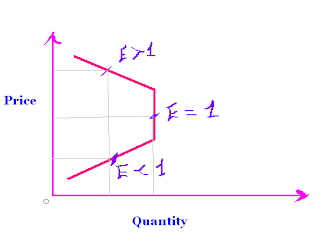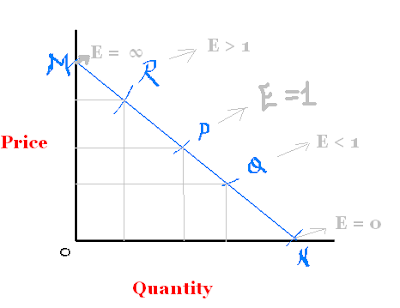## >> Thursday, May 21, 2009Definition of Elasticity of demand

Elasticity of demand may be defined as percentage changes in demand divided by percentage changes in prices . This is the simple measurement of the changes in the value of demand , if any changes happens in prices . Suppose if Ram purchased fruit 10 kgs at Rs. 5 per kg . If the price of fruit increase by Rs. 1 and demand of Ram decreases by 8 kgs it means demand of Ram is more elastic and its value can be found by elasticity of demand formula

Formula for calculating elasticity of demand

= % change in demand / % change in prices

Degrees of elasticity of demand

1. Perfect elasticity of demand

Perfect elasticity of demand can explain as highest changes in the value of demand due to changes in price if a little changes in price , the infinite changes will be done in demand . In other words except above definition , it is just define as E = ∞

2. Unitary elasticity of demand

If changes in prices and demand with equal proportion , then its elasticity of demand is equal to one .

Suppose Ram purchased one ice cream at rs. 2 and if the price will decrease by rs. 1 then the demand becomes more by purchasing one more quantity of ice cream. Then E = 1

3. Less elasticity of demand

Less elasticity of demand is less than one . E <1>

4. More elasticity of demand

If any changes in price will change the demand with high proportion , then this degree is called more elasticity of demand . It is E > 1

Methods of measuring Elasticity of demand

There are three methods for measuring elasticity of demand .

1. Total outlay or total expenditure method

In this method , we measure elasticity of demand on the basis of total expenditure or total outlay . Total expenditure is the product of price and quantity purchased . If total expenditure will increase by increasing price , it is less elastic demand , If total expenditure does not change by changing demand . This is unitary elastic demand . If total expenditure decreased , if prices increases , then this is high elastic demand . We can also show elasticity of demand on graph paper under total outlay method .2. Point Method

Under this method , we measure elasticity of demand on the based points on a graph . We know the formula for calculating elasticity of demand that is

= change in demand / change in prices X original price / original quantity

But when it show on graph its formula is

Lower part of demand curve / upper part of demand curve

Unitary elasticity of demand = PN / PM = 1

Less elasticity of demand = QN/ QM = E<>
More elasticity of demand = RN/RM = E > 13. Arc method
Under this method , we can calculate elasticity of demand between two points in any demand curve . The following formula will apply
E = change in demand / change in prices X ( original price + new price )/2 / ( original quantity + new quantity ) / 2|